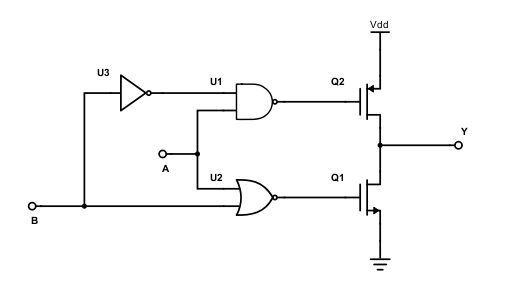# Interview Questions for Standard Cell Design Engineer Library Design Engineer Job Profile

## INTERVIEW QUESTIONS LIBRARY DESIGN

In this post we are providing some typical interview questions asked when you are looking giving interview for standard cell design position or library design engineer position or related job profile targeting standard cell related job profile.

These questions are mainly asked to freshers (NCGs) or 1-2 years of experience candidates who are going to start their journey in Standard Cell Design field.Questions

1. Draw Inverter Voltage Transfer Characteristics and clearly mention all the regions with details.
2. What do you mean by delay? (Give Definition)
3. Why do we take 50% of input while measuring delay?
4. What are different power in CMOS circuit?
5. What are the different components of Leakage current?
6. What is short circuit current?
7. Does leakage current flow when transistor is on?
8. What is the difference between a latch and a flipflop?
9. Draw 2:1 MUX using logic gate.
10. How can you draw a flipflop using MUX?
11. Calculate setup and hold time of the circuit above.
12. Why slew is defined for 20%-80% or 10%-90 %?
13. Mention all the factors that affect the delay of a circuit.
14. Let’s consider the below situation where S means slew and C means capacitor.
1. S1>>S2>>S3
2. C1>>C2>>C3

For which combination of slew and capacitor you will get (i) maximum delay (ii) minimum delay

1. How do slew and capacitor affects the power as per above question?
2. How do power calculation tools such as PT-PX calculates power of VLSI circuit?
3. Can propagation delay be negative?
4. What is functionality of the below circuit?Ans: Tristate Buffer. Here, B input is the control input of the tri-state buffer. When B=0, it acts like a simple buffer. If B=1, both NMOS and PMOS is off irrespective of A value. Now, if B=0, both the PMOS and NMOS gate inputs gate A’. So, it’s like A’ is fed to an inverter whose output is simply A.
If you  draw the truth table it will be something like below.

 A B Y 0 0 0 0 1 Z 1 0 1 1 1 Z

If you have any questions regarding the above, please comment below.